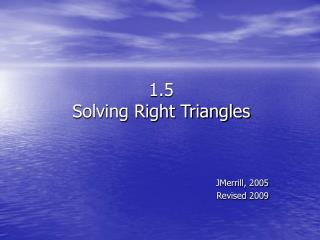DownloadDownload Presentation1.5 Solving Right Triangles

1.5 Solving Right Triangles

Télécharger la présentation1.5 Solving Right Triangles

- - - - - - - - - - - - - - - - - - - - - - - - - - - E N D - - - - - - - - - - - - - - - - - - - - - - - - - - -
Presentation Transcript

1. 1.5Solving Right Triangles JMerrill, 2005 Revised 2009

2. Recall from Last Week SOH-CAH-TOA • Sine: abbreviation: sin = • Cosine: abbreviation: cos = • Tangent: abbreviation: tan = • These are the ratios of 2 sides.

3. Evaluating with a Calculator When • Mode is in degrees (for now!) • Solve: • cos 41o = tan 87o = • sin 18o = cos 180o = • These are still the ratios of 2 sides—in decimal form.

4. A plane is one mile above sea level when it begins to climb at a constant angle of 2 for the next 70 ground miles. How far above sea level is the plane after its climb? So, about 3 ½ miles total. Application—Finding the Length of a Side 2o x 70

5. You do: • A 16-foot ladder is propped against a building. The angle it forms with the ground measures 55. How far up the side of the building does the ladder reach? 16 x 55o

6. To find the measure of an angle you must take the inverse of the function. The inverse tangent, for example, is written tan-1. On your calculator, you push 2nd tan.Anytime you are looking for the angle, you push the 2nd button! θ is the Greek symbol for theta (lower case). It will be used to represent an angle in degrees. 553 100 Finding an Angle θ

7. Angle of Elevation • Angle of elevation is the angle formed by the horizontal line of sight to an object that is above the horizontal line.

8. Angle of Depression • Angle of depression is the angle formed by the horizontal line of sight to an object that is below the horizontal line.

9. You do:

10. How far are you from the ground? How tall is the pole? Solutions You are approximately 20.1 feet from the ground. From your position to the top of the pole is approximately 34.97 feet. Therefore, 20.1 + 34.97 = 55.07. Thus, the pole is about 55.07 feet tall.

11. Navigation • Direction is often given as a measure of an acute angle with respect to the north-south vertical line. A bearing (or heading) of N 20o E means that the plane/ship is bearing 20o to the east of north. • So, S 48o W means? • 48o to the west of south

12. Example • A jet takes off on a bearing of N 28o E, flies 5 miles, then makes a left (90o) turn and flies 12 miles. If the control tower operator wanted to locate the plane, what bearing would she use?